# 桠枝

Twigbranch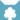天族 (Q632)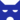影族 (Q628)[临时][避难]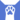反抗者 (Q4414)天族 学徒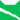雷族 学徒雷族 武士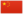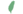小桠 Twigkit  小嫩枝
 桠爪 Twigpaw  嫩枝掌
 桠枝 Twigbranch  嫩枝杈

Q3496：雷族母猫，原天族猫，自《学徒探索》登场的角色

## 细节

### 外貌

• 她是一只身形苗条、:185皮毛蓬松:17光泽、:279绿色眼睛:猫物表的灰色短毛母猫，:267一只耳尖切裂。:352
• 她的外貌和父亲鹰翅极为相似，:57并被描述和他拥有相同颜色的皮毛。:53:6章此外，她的绿色眼睛的颜色深浅和母亲卵石光的完全相同。:268

## 登场书目

• 学徒探索 (Q170)[配角][首次登场🐱]
• 雷影交加 (Q171)[视角人物]
• 天空破碎 (Q172)[视角人物]
• 极夜无光 (Q173)[视角人物]
• 烈焰焚河 (Q174)[视角人物]
• 风暴来袭 (Q175)[视角人物]
• 迷失群星 (Q3563)[配角]
• 静默冰融 (Q3579)[配角]
• 叠影重障 (Q3624)[配角]
• 暗由心生 (Q4183)[配角]
• 无星之地 (Q4385)[配角]
• 迷雾明光 (Q4621)[对话提及]
• 河翻浪涌 (Q4643)[配角]
• 天穹将倾 (Q4799)[配角]
• 虎心的阴影 (Q185)[对话提及]
• 松鼠飞的希冀 (Q3561)[配角]
• 灰条的誓言 (Q4057)[配角]
• 一星的告解 (Q4826)[配角]
• 褐皮的族群 (Q3566)[猫物闲角]
• 卵石光的幼崽 (Q3626)[配角]
• 黛西的至亲 (Q3629)[对话提及]
• 斑毛的反抗 (Q4337)[配角]

1. 因放逐而离开

## 参考文献

这篇文章基于CC BY-SA 3.0许可使用了猫武士维基（英语）Twigbranch一文中的部分内容。